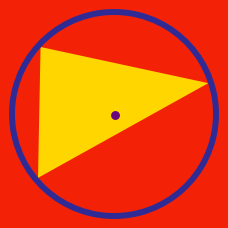Geometry

Triangle Centers: Level 3 Challenges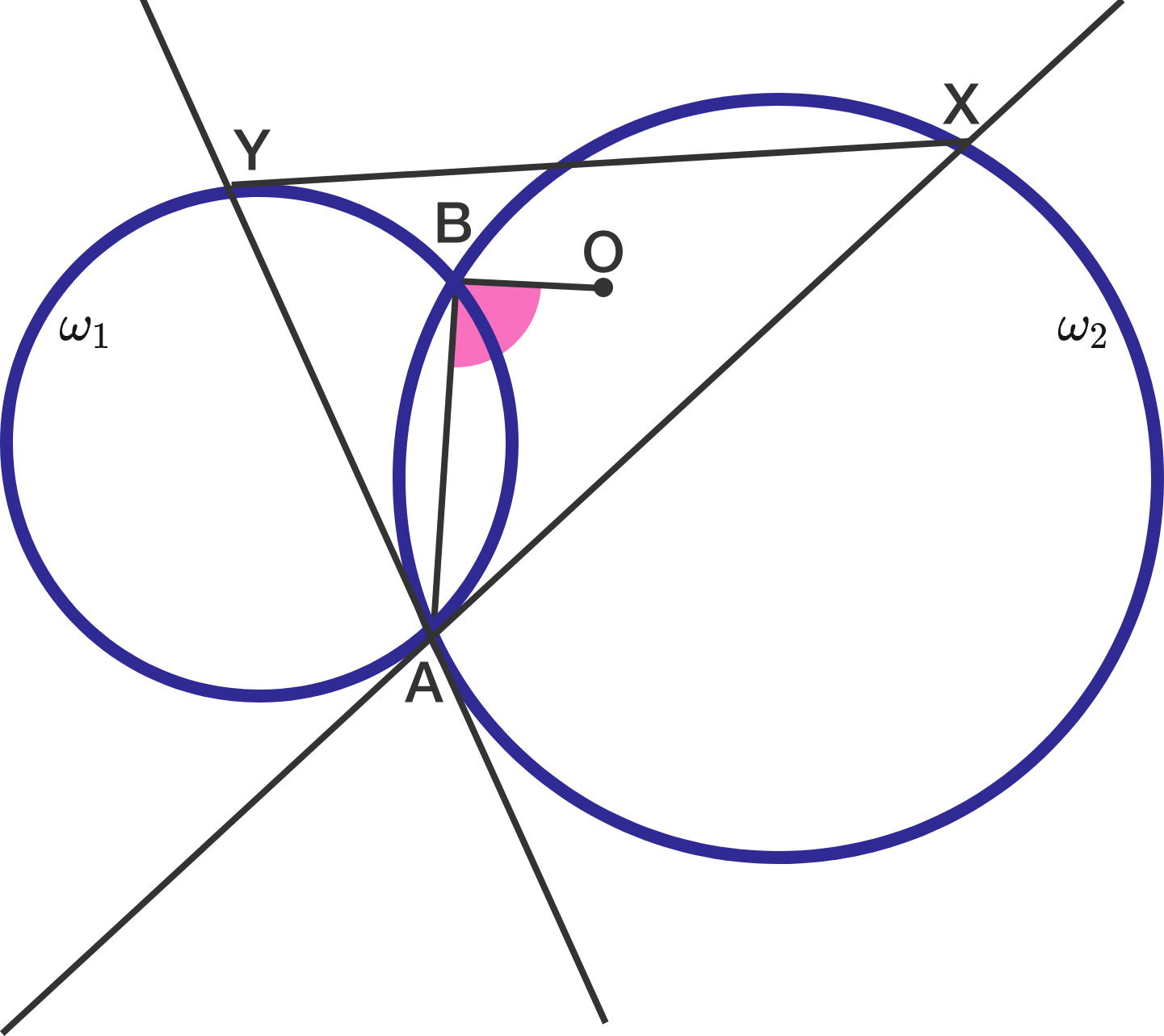Two circles $\omega_1$ and $\omega_2$ intersect at points $A$ and $B$. The tangent to $\omega_1$ passing through $A$ intersects $\omega_2$ at $X$. The tangent to $\omega_2$ passing through $A$ intersects $\omega_1$ at $Y$. Let $O$ be the circumcenter of $\triangle XAY$. Then what is the measure of $\angle OBA$ in degrees?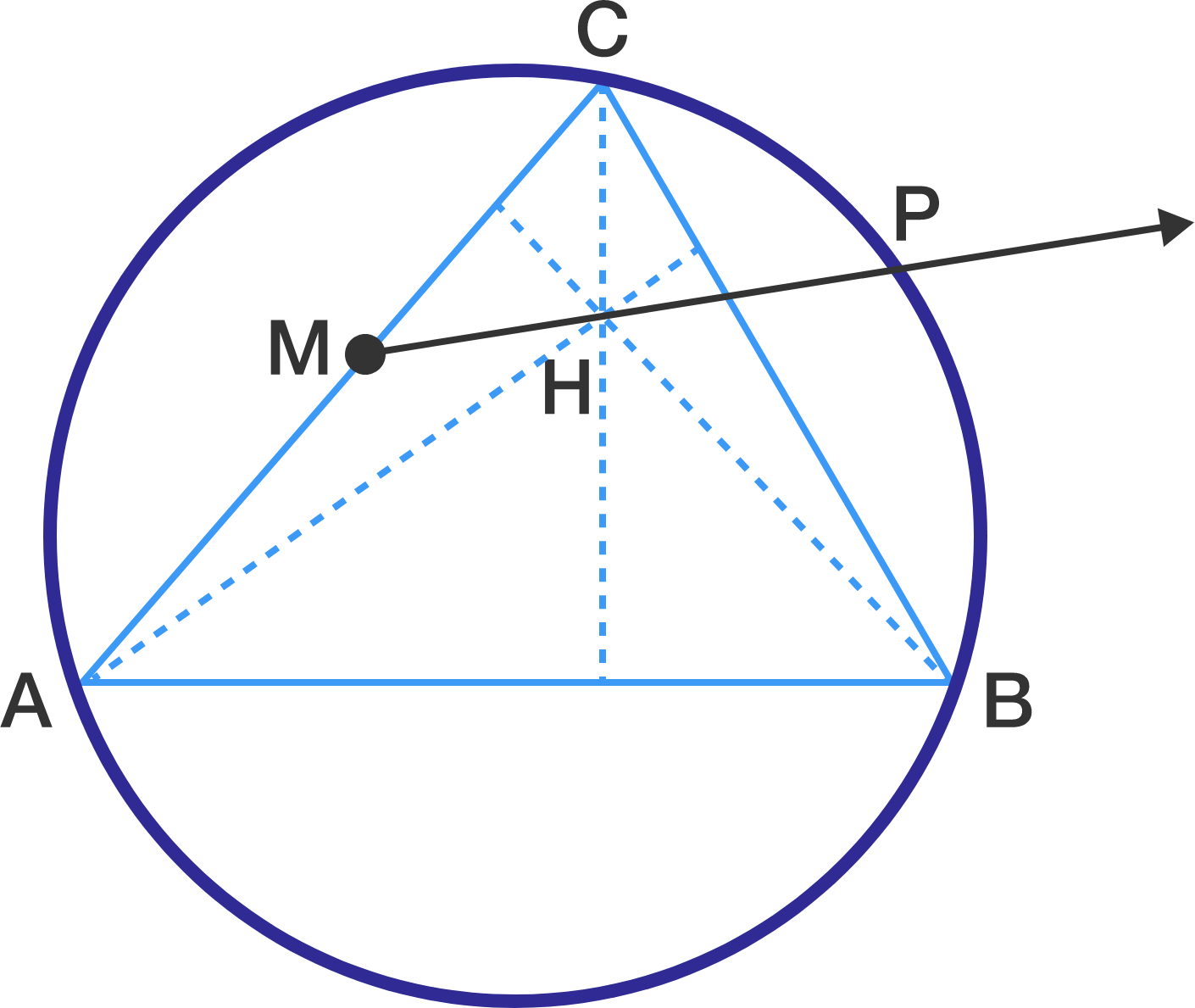Let $ABC$ be an acute triangle with $AB > BC$. Let $H$ be the orthocenter (intersection of altitudes) of this triangle and $M$ be the midpoint of $AC$. The ray $\overrightarrow{M H}$ intersects the circumcircle of triangle $ABC$ at point $P$, where $P$ belongs to the minor arc $BC$. It is known that $\angle ABP=90^{\circ}$, $MH=5$ and $HP=16$. Find the length of $BC$.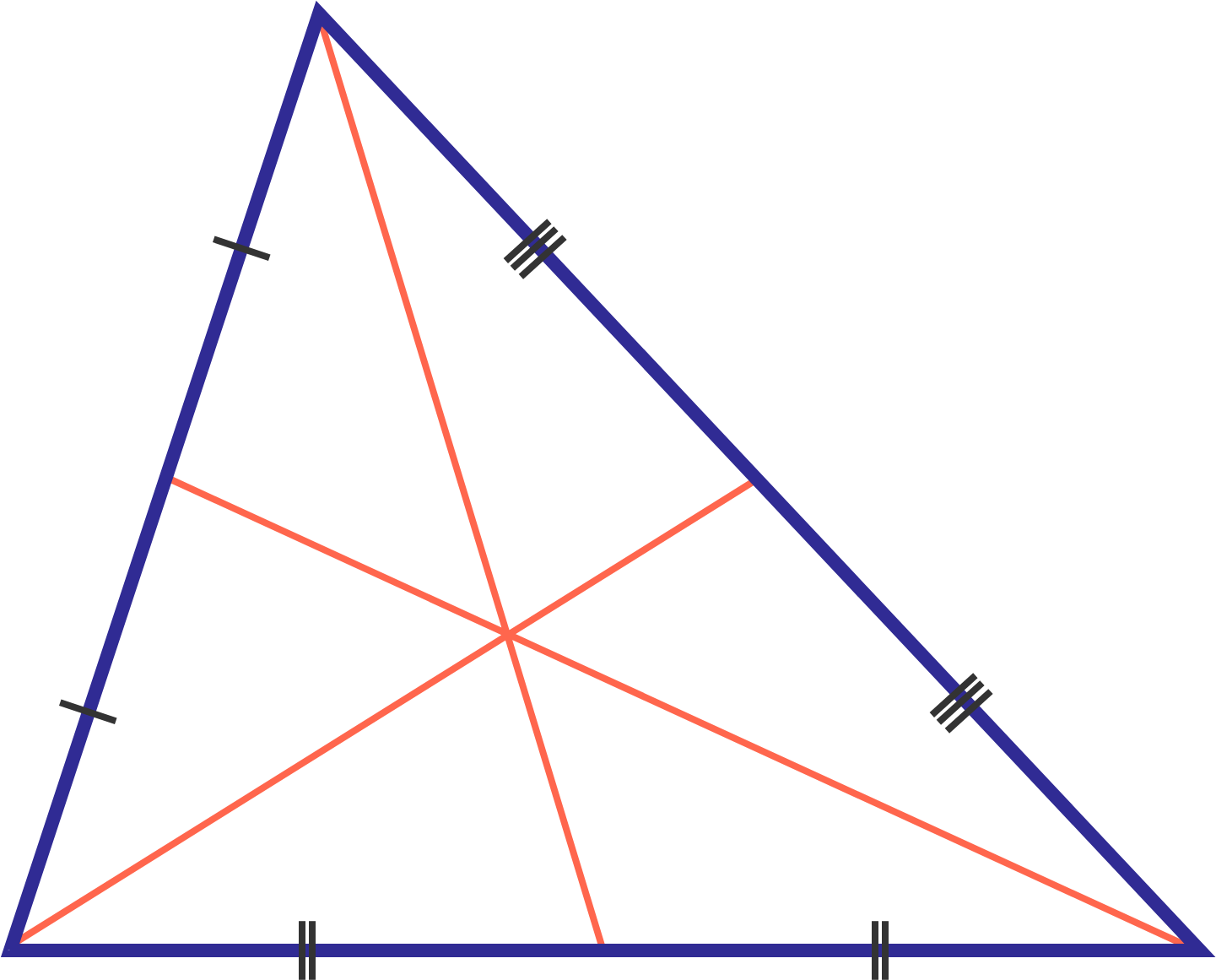If the medians of triangle $ABC$ intersect at $O$, then evaluate $\frac{AB^2+BC^2+AC^2}{OA^2+OB^2+OC^2}$.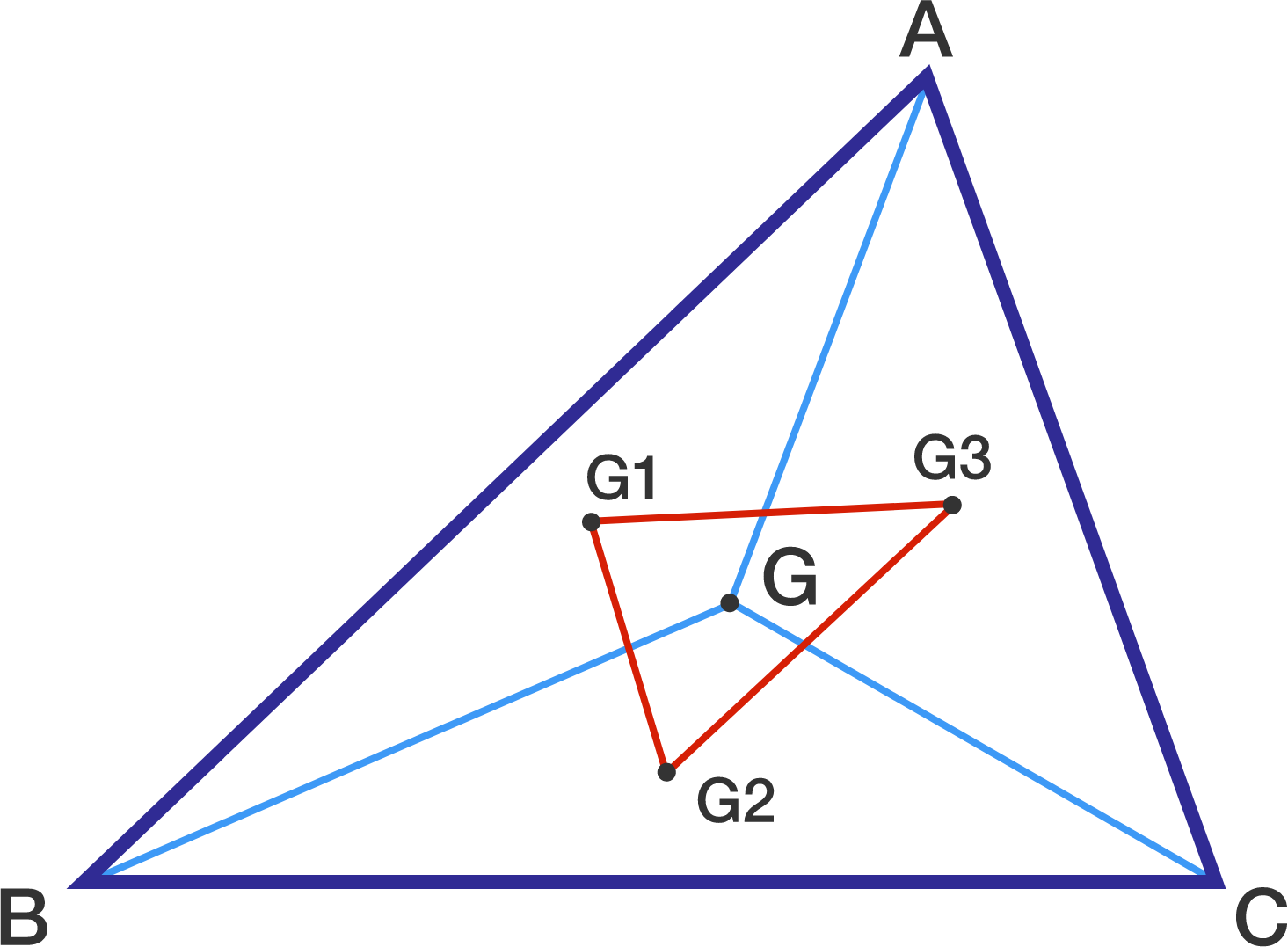Triangle $ABC$ has centroid $G$. Triangles $ABG$, $BCG$, and $CAG$ have centroids $G_1,G_2,G_3$ respectively.

The value of $\dfrac{[G_1G_2G_3]}{[ABC]}$ can be represented by $\dfrac{p}{q}$, for positive coprime integers $p,q$.

Find $p+q$.

$\text{Details and Assumptions:}$

$[ABCD]$ denotes the area of $ABCD$.

How many triangles $ABC$ are there with integer side lengths such that the area of the triangle formed by joining the orthocenter, the circumcenter and the centroid of $\triangle ABC$ is $44$ square units?

Details and assumptions:

The orthocenter of $ABC$ is the point at which the altitudes of $ABC$ intersect.

The circumcenter of $ABC$ is the point which is equidistant from $A$, $B$ and $C$.

The centroid of $ABC$ is the point at which the medians of $ABC$ intersect.

×